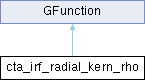GammaLib  1.7.0.dev

Kernel for radial model zenith angle integration of IRF. More...

#include <GCTAResponse_helpers.hpp>## Public Member Functions

cta_irf_radial_kern_rho (const GCTAResponseIrf *rsp, const GModelSpatialRadial *model, const double &zenith, const double &azimuth, const GEnergy &srcEng, const GTime &srcTime, const GEnergy &obsEng, const double &zeta, const double &lambda, const double &omega0, const double &delta_max, const int &iter)

double eval (const double &rho)
Kernel for radial model zenith angle integration of Irf. More...Public Member Functions inherited from GFunction
GFunction (void)
Void constructor. More...

GFunction (const GFunction &function)
Copy constructor. More...

virtual ~GFunction (void)
Destructor. More...

GFunctionoperator= (const GFunction &function)
Assignment operator. More...

## Protected Attributes

const GCTAResponseIrfm_rsp
CTA response. More...

double m_zenith
Zenith angle. More...

double m_azimuth
Azimuth angle. More...

GEnergy m_srcEng
True photon energy. More...

GTime m_srcTime
True photon time. More...

GEnergy m_obsEng
Measured event energy. More...

double m_zeta
Distance model centre - measured photon. More...

double m_cos_zeta
Cosine of zeta. More...

double m_sin_zeta
Sine of zeta. More...

double m_lambda
Distance model centre - pointing. More...

double m_cos_lambda
Cosine of lambda. More...

double m_sin_lambda
Sine of lambda. More...

double m_omega0
Azimuth of pointing in model system. More...

double m_delta_max

double m_cos_delta_max
Cosine of maximum PSF radius. More...

int m_iter
Integration iterations. More...Protected Member Functions inherited from GFunction
void init_members (void)
Initialise class members. More...

void copy_members (const GFunction &function)
Copy class members. More...

void free_members (void)
Delete class members. More...

## Detailed Description

Kernel for radial model zenith angle integration of IRF.

Kernel for radial model zenith angle integration.

This class implements the integration kernel $$K(\rho)$$ for the integration

$\int_{\rho_{\rm min}}^{\rho_{\rm max}} K(\rho | E, t) d\rho$

of radial spatial models. The eval() method computes

$K(\rho | E, t) = \sin \rho \times S_{\rm p}(\rho | E, t) \times \int_{\omega_{\rm min}}^{\omega_{\rm max}} IRF(\rho, \omega) d\omega$

where

• $$S_{\rm p}(\rho | E, t)$$ is the radial model,
• $$IRF(\rho, \omega)$$ is the IRF
• $$\rho$$ is the distance from the model centre, and
• $$\omega$$ is the azimuth angle is the position angle with respect to the connecting line between the model centre and the observed photon arrival direction.

This class implements the integration kernel $$K(\rho)$$ for the integration

$\int_{\rho_{\rm min}}^{\rho_{\rm max}} K(\rho | E, t) d\rho$

of radial spatial models. The eval() method computes

$K(\rho | E, t) = \sin \rho \times S_{\rm p}(\rho | E, t) \times \int_{\omega} PSF(\rho, \omega) d\omega$

where $$S_{\rm p}(\rho | E, t)$$ is the radial model, $$PSF(\rho, \omega)$$ is the point spread function, $$\rho$$ is the distance from the model centre, and $$\omega$$ is the position angle with respect to the connecting line between the model centre and the observed photon arrival direction.

Definition at line 175 of file GCTAResponse_helpers.hpp.

## Constructor & Destructor Documentation

 cta_irf_radial_kern_rho::cta_irf_radial_kern_rho ( const GCTAResponseIrf * rsp, const GModelSpatialRadial * model, const double & zenith, const double & azimuth, const GEnergy & srcEng, const GTime & srcTime, const GEnergy & obsEng, const double & zeta, const double & lambda, const double & omega0, const double & delta_max, const int & iter )
inline

Definition at line 177 of file GCTAResponse_helpers.hpp.

## Member Function Documentation

 double cta_irf_radial_kern_rho::eval ( const double & rho )
virtual

Kernel for radial model zenith angle integration of Irf.

Parameters
 [in] rho Zenith angle with respect to model centre [radians].

Computes the kernel

$K(\rho | E, t) = \sin \rho \times S_{\rm p}(\rho | E, t) \times \int_{\omega_{\rm min}}^{\omega_{\rm max}} IRF(\rho, \omega) d\omega$

for the zenith angle integration of radial models.

Implements GFunction.

Definition at line 331 of file GCTAResponse_helpers.cpp.

## Member Data Documentation

protected

Azimuth angle.

Definition at line 211 of file GCTAResponse_helpers.hpp.

Referenced by eval().

protected

Definition at line 223 of file GCTAResponse_helpers.hpp.

Referenced by eval().

protected

Cosine of lambda.

Definition at line 219 of file GCTAResponse_helpers.hpp.

Referenced by eval().

protected

Cosine of zeta.

Definition at line 216 of file GCTAResponse_helpers.hpp.

Referenced by eval().

protected

Definition at line 222 of file GCTAResponse_helpers.hpp.

Referenced by eval().

protected

Integration iterations.

Definition at line 224 of file GCTAResponse_helpers.hpp.

Referenced by eval().

protected

Distance model centre - pointing.

Definition at line 218 of file GCTAResponse_helpers.hpp.

Referenced by eval().

protected

Definition at line 209 of file GCTAResponse_helpers.hpp.

Referenced by eval().

protected

Measured event energy.

Definition at line 214 of file GCTAResponse_helpers.hpp.

Referenced by eval().

protected

Azimuth of pointing in model system.

Definition at line 221 of file GCTAResponse_helpers.hpp.

Referenced by eval().

protected

CTA response.

Definition at line 208 of file GCTAResponse_helpers.hpp.

Referenced by eval().

protected

Sine of lambda.

Definition at line 220 of file GCTAResponse_helpers.hpp.

Referenced by eval().

protected

Sine of zeta.

Definition at line 217 of file GCTAResponse_helpers.hpp.

Referenced by eval().

protected

True photon energy.

Definition at line 212 of file GCTAResponse_helpers.hpp.

Referenced by eval().

protected

True photon time.

Definition at line 213 of file GCTAResponse_helpers.hpp.

Referenced by eval().

protected

Zenith angle.

Definition at line 210 of file GCTAResponse_helpers.hpp.

Referenced by eval().